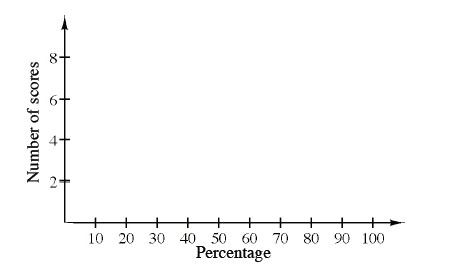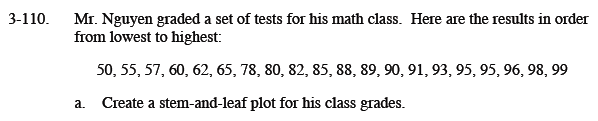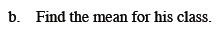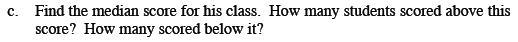### Home > MC1 > Chapter 3 > Lesson 3.4.2 > Problem3-110

3-110.
1. . Mr. Nguyen graded a set of tests for his math class. Here are the results in order from lowest to highest:
50, 55, 57, 60, 62, 65, 78, 80, 82, 85, 88, 89, 90, 91, 93, 95, 95, 96, 98, 99 Homework Help ✎

1. Create a stem-and-leaf plot for his class grades.

2. Find the mean for his class.

3. Find the median score for his class. How many students scored above this score? How many scored below it?To review stem-and-leaf plots, refer to the Math Notes box in Lesson 1.3.1.See the Math Notes box in Lesson 1.3.3.The median score in Mr. Nguyen is 86.5.
Can you find how many scores are above and below the median?